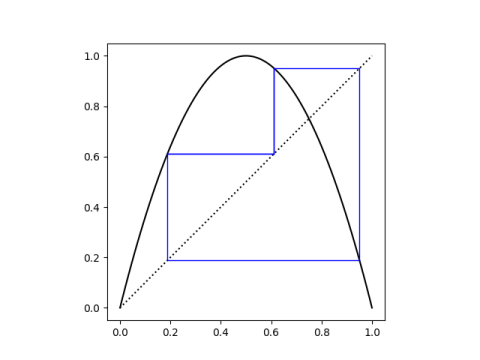The previous post Does chaos imply period 3? ended with looking at a couple cubic polynomials whose roots have period 3 under the mapping f(x) = 4x(1-x). These are

64 x³ – 112 x² + 56 x – 7

and

64 x³ – 96 x² + 36 x – 3.

I ended the post by saying you could find their roots numerically using the NSolve function in Mathematica.

What if you wanted to find the roots exactly? You could try using the NSolve function rather than the NSolve solve function, but you won’t get anything back. Doesn’t Mathematica know how to solve a cubic equation? Yes it does, and you can force it to use the cubic equation by using the ToRadicals function.

If you apply ToRadicals to the roots, Mathematica will give you the roots in radical form, with expressions involving complex numbers. For example, here’s the smallest root of the first polynomial:But the roots of these polynomials are clearly real as you can see from their graphs.

.If we didn’t have access to a graph, we could show that the roots are real and distinct by computing the discriminant and seeing that it is positive.

## Casus irreducibilis

The polynomials have degree less than 5, so their roots can be expressed in terms of radicals. And the roots are real. But they cannot be expressed in terms of real radicals!

This an example of the “casus irreducibilis.” Most cubic equations with real roots cannot be solved using real radicals. The casus irreducibilis theorem says that the roots of a cubic polynomial can be written in terms of real radicals if and only if one of the roots is rational.

This says there’s a sort of excluded middle ground: the roots cannot involve cubic roots with real numbers. They are either simpler or more complex. If there is a rational root, you can factor it out and find the other two roots with the quadratic equation, i.e. only using square roots, not cubic roots. But if there is no rational root, expressing the roots in terms of radicals requires cube roots and complex numbers.

## Our polynomials

The rational root test says that if a polynomial has a rational root p/q then q must be a factor of the leading coefficient and p must be a factor of the constant term. So in our first cubic

64 x³ – 112 x² + 56 x – 7

any rational root must have denominator 1, 2, 4, …, 64 and numerator either 1 or 7. We can check all the possibilities and see that none of them are zeros.

Similarly the only possible rational roots of

64 x³ – 96 x² + 36 x – 3

must have denominator 1, 2, 4, …, 64 and numerator either 1 or 3, and none of them are zeros.

So the roots of our cubic polynomials can be expressed in terms of radicals, but not without complex numbers, even though the roots are all real.

## Unstable orbit

The reason we were interested in these two polynomials is that we were looking for orbits of period 3 under iterations of 4x(1-x). And we found them. But they form an unstable orbit.

If we make a cobweb plot of the iterations starting at any one of the roots, it appears that multiple applications of 4x(1-x) just rotate between the three roots.But with a few more iterations—the plot below shows 100 iterations—we can see that these points are not stable. Unless you start exactly on one of the roots, which you cannot since they’re irrational, you will wander away from them.## 3 thoughts on “Real radical roots”

1. This is an interesting point. To add one nuance to this: Some cycles can remain periodic because irrational numbers are represented by truncated floating-point numbers.

The opposite point can also be made: If the iteration is run with any starting value, it will eventually fall into a period due to truncation and roundoff. With 64-bit numbers this may indeed take very many iterations and involve long periods, and it is easier to detect with 32-bit numbers.

2. Jonathan

I think you need to add “We will be considering polynomials with integer coefficients (or just rational coefficients, which turns out to be equivalent if all you care about is finding their roots)” somewhere at the beginning, no?

3. Vaguely related (dual?) inquiry, find the value(s) of u so that the function f(x)=ux(x-1) has a superattracting orbit of period 3.

If memory serves, there is a unique solution for u, and x=1/2 must be in the orbit, and u can be solved in closed form.